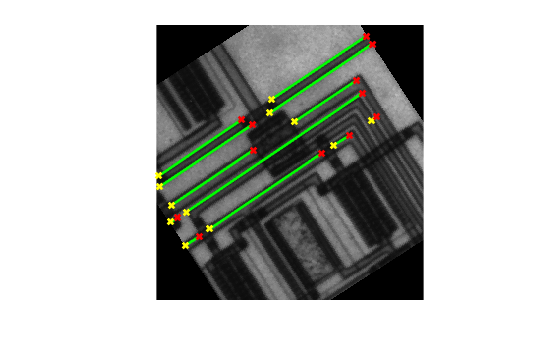Main Content

## Hough Transform

The Image Processing Toolbox™ supports functions that enable you to use the Hough transform to detect lines in an image.

The hough function implements the Standard Hough Transform (SHT). The Hough transform is designed to detect lines, using the parametric representation of a line:

rho = x*cos(theta) + y*sin(theta)

The variable rho is the distance from the origin to the line along a vector perpendicular to the line. theta is the angle between the x-axis and this vector. The hough function generates a parameter space matrix whose rows and columns correspond to these rho and theta values, respectively.

After you compute the Hough transform, you can use the houghpeaks function to find peak values in the parameter space. These peaks represent potential lines in the input image.

After you identify the peaks in the Hough transform, you can use the houghlines function to find the endpoints of the line segments corresponding to peaks in the Hough transform. This function automatically fills in small gaps in the line segments.

### Detect Lines in Images Using Hough

This example shows how to detect lines in an image using the Hough transform.

Read an image into the workspace and, to make this example more illustrative, rotate the image. Display the image.

I = imread('circuit.tif'); rotI = imrotate(I,33,'crop'); imshow(rotI)Find the edges in the image using the edge function.

BW = edge(rotI,'canny'); imshow(BW);Compute the Hough transform of the binary image returned by edge.

[H,theta,rho] = hough(BW);

Display the transform, H, returned by the hough function.

figure imshow(imadjust(rescale(H)),[],... 'XData',theta,... 'YData',rho,... 'InitialMagnification','fit'); xlabel('\theta (degrees)') ylabel('\rho') axis on axis normal hold on colormap(gca,hot)Find the peaks in the Hough transform matrix, H, using the houghpeaks function.

P = houghpeaks(H,5,'threshold',ceil(0.3*max(H(:))));

Superimpose a plot on the image of the transform that identifies the peaks.

x = theta(P(:,2)); y = rho(P(:,1)); plot(x,y,'s','color','black');Find lines in the image using the houghlines function.

lines = houghlines(BW,theta,rho,P,'FillGap',5,'MinLength',7);

Create a plot that displays the original image with the lines superimposed on it.

figure, imshow(rotI), hold on max_len = 0; for k = 1:length(lines) xy = [lines(k).point1; lines(k).point2]; plot(xy(:,1),xy(:,2),'LineWidth',2,'Color','green'); % Plot beginnings and ends of lines plot(xy(1,1),xy(1,2),'x','LineWidth',2,'Color','yellow'); plot(xy(2,1),xy(2,2),'x','LineWidth',2,'Color','red'); % Determine the endpoints of the longest line segment len = norm(lines(k).point1 - lines(k).point2); if ( len > max_len) max_len = len; xy_long = xy; end end % highlight the longest line segment plot(xy_long(:,1),xy_long(:,2),'LineWidth',2,'Color','red');Download ebook